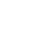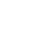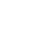# Energy of Motion

Physics is the science that studies motion and matter and how both work with forces and energy. It is a really big subject that has a lot of different “branches” of scientific study that include motion, sound, waves, electricity, light, sound, and even astronomy.

This science covers the tiniest particles and atoms all the way to the largest objects in the universe. Sir Isaac Newtown developed the three laws of motion to help to explain how things work scientifically:

• First Law of Motion states that an object that is in motion will continue to move in the same direction and speed unless some force or forces act upon it.
• Second Law of Motion states that the greater an object’s mass, the more force it will take to accelerate that object.
• The Third Lawn of Motion states that for every action, there is an equal and opposite reaction. This is involves the energy of motion and means that there are always two of the forces that are the same. An example would be the amount of force it took you to pull your foot back to kick a ball. One force is the preparation to kick and the other force is actually kicking the ball.

There are a lot of different types of energy and scientists use the idea of energy to explain why and how things behave like they do. We may have heard about solar energy, electrical energy, chemical energy, and even nuclear energy.

It’s important to know that we can’t touch or hold energy, it’s just a way to help us understand how things work. Energy is measured in units called “joules.”Kinetic Energy (KE) is found in objects that are moving. An example of kinetic energy can be found when a car or roller coaster is moving. Another example is the water running in a brook.

Potential energy (PE) is energy that is “stored”. An example of this might be a ball sitting on the ground. When you pick it changes the energy that it has when you hold it over your head and then increases the stored energy when you throw it. You become the “force” that releases the potential energy.

### Rubber bands and springs

A rubber band sitting on a table is pretty boring looking. But it can be picked up and then stretched and that changes everything. Once you apply the “work” involved in stretching the rubber band, it increases the potential energy and stores it. The energy is released when you release the rubber band.

Believe it or not, there is an entire section of physics devoted just to the study of “springs.” If you look at a spring it is pretty boring, but once you push on that spring you are exerting a “force” that alters the coil arrangements and stores energy. When you let up the energy is released.

Each of these examples is using a force to put energy in and then seeing a reaction as energy comes out.

### Gases can store energy

Gases act like a kind of elastic in that they can expand or compress when pressure increases or decreases. Gas molecules can store the energy and then let it out to do some kind of work. An example of this is when the wind blows and hits a windmill.

The wind is due to the atmospheric differences in pressure. The turning of the windmill is a kind of work. A turning windmill can use the energy to move another object that grinds wheat or create flowing water for drinking or the fields.

Motion energy is also called mechanical energy. This is the energy that is stored in moving objects and as the object moves faster, more energy is stored. Motion energy is the total of the kinetic and potential energy in an object that is used to accomplish the work.

### Q&A:

How many laws of motion were defined by Sir Isaac Newton?
3

What is the unit of measure used for energy?
joules

What is Kinetic Energy?
energy found in objects that are moving

What is Potential Energy?
energy that is stored

What is another name for motion energy?
mechanical energy

What is motion energy?
the total of the kinetic and potential energy in an object that is used to accomplish the work

•History
•Science
•Geography
•Biography
•US History